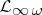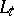# Characterization theorems for logics

First-order logic (cf. also Logical calculus) is well-suited for mathematics, e.g.:

1) There is a sound and complete proof calculus (completeness theorem). The decidability of many theories has been proven using the completeness theorem. (Cf. also Completeness (in logic); Sound rule.)

2) There is a system of first-order logical axioms for set theory (e.g., ZFC) that serves as a basis for mathematics.

3) There is a balance between syntax and semantics, e.g., implicitly definable concepts are explicitly definable (Beth's theorem; cf. also Beth definability theorem).

4) Semantic results such as the compactness theorem and the Löwenheim–Skolem theorem are valuable model-theoretic tools and lead to an enrichment of mathematical methods. Mainly in the period from 1950 to 1970, much effort was spent in finding languages which strengthen first-order logic but are still simple enough to yield general principles which are useful in investigating and classifying models. In particular, taking into account the situation for first-order logic, many logicians attempted to find logics satisfying analogues of the theorems mentioned above. However, results due to P. Lindström [a3] limit this search. Or, to state it more positively, Lindström proved the following characterization theorems for first-order logic:

First-order logic is a maximal logic with respect to expressive power satisfying the compactness theorem and the Löwenheim–Skolem theorem.

First-order logic is a maximal logic satisfying the completeness theorem and the Löwenheim–Skolem theorem.

These results were the starting point for investigations trying to order the diversity of extensions of first-order logic, for a systematic study of the relationship between different model-theoretic properties of logics, and for a search for further characterizations theorems for first-order and other logics.

Most of the results obtained can be found in [a1]. Two characterization theorems obtained for other logics are:

a)is a maximal bounded logic with the Karp property [a2];

b) for topological structures, the logicof "invariant sentences" is a maximal logic satisfying the compactness theorem and the Löwenheim–Skolem theorem [a4].

How to Cite This Entry:
Characterization theorems for logics. Joerg Flum (originator), Encyclopedia of Mathematics. URL: http://encyclopediaofmath.org/index.php?title=Characterization_theorems_for_logics&oldid=18155
This text originally appeared in Encyclopedia of Mathematics - ISBN 1402006098